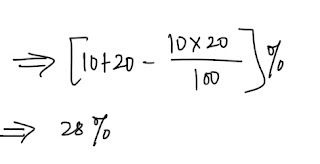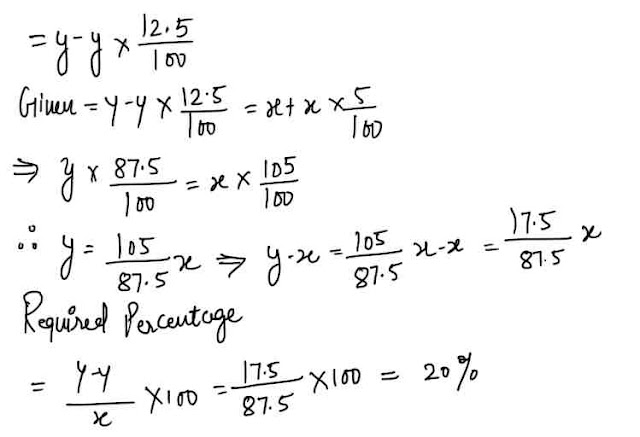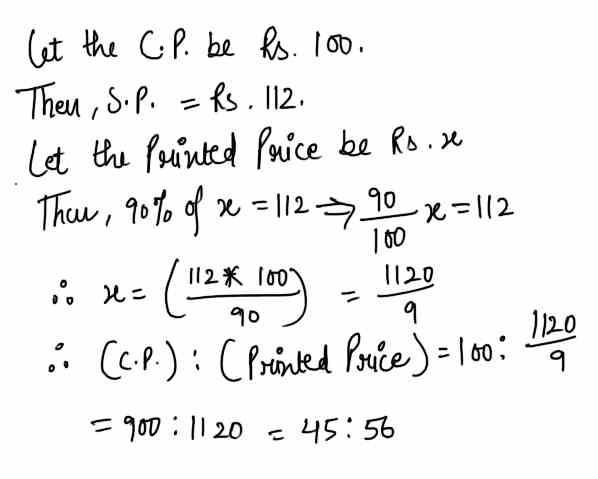# Profit and Loss - 10 Important Questions for SBI Clerk Exam#### Ques 1.

A dishonest shopkeeper professes to sell his goods at the cost price but use faulty measure. His 1 kg weight measures 950 grams only. find his gain percent.
Ans 1.#### Ques 2.

Find the single discount which is equivalent to a successive discounts of 50% and 40%.
Ans 2.#### Ques 3.

Find the single discount which is equivalent to three successive discount 10%, 20% and 30%.
Ans 3. Here first to all we will determine the single discount, which is equivalent to two successive discounts of 10% and 20%.'Now, we will find a single discount which is equivalent to two successive discounts of 28% and 30%.#### Ques 4.

A dozen pairs of gloves quoted at Rs. 80 are available at a discount of 10%. Find how many pairs of gloves can be bought for Rs. 24.
(a) 4
(b) 5
(c) 6
(d) 8
Ans 4.#### Ques 5.

How much percent more than the cost price should a shopkeeper mark his goods, so that after allowing a discount of 12.5% he should have a gain 5% on his outlay ?
(a) 9.375
(b) 16.66%
(c) 20%
(d) 25%
Ans 5.  Let the cost price be x Rs. and printed price be y Rs.
Hence, price after giving a discount of 12.5 %#### Ques 6.

Two mixers and one T.V. cost Rs. 7000, while two T.V.'s and a mixer cost Rs. 9800.The value of one T.V. is
(a) Rs 2800
(b) Rs. 2100
(c) Rs. 4200
(d) Rs. 8400
Ans 6. Let C.P. of mixer be Rs. x and that of a T.V. be Rs. y.
Then, 2x + y = 7000
and X + 2y  = 9800
Multiplying equation (ii) by 2 and sub-tracting  equation (i) from it, we get
3 y  = 19600-7000 = 12600 or y = 4200
.. C.P. of as T.V. = Rs. 4200

#### Ques 7.

A fruit seller has 24kg of apples. He sells a part of these at 20% gain and the balance at a loss 5%. If on the whole the earn a profit of 10%, the amount of apples sold at loss is :
(a) 6 kg
(b) 4.6 kg
(c) 9.6 kg
(d) 11.4 kg
Ans 7. Suppose, the quantity  sold at  a loss be x kg and let C.P. per kg Re. 1
Total C.P. = Rs. 24.#### Ques 8.

A shopkeeper earns a profit of 12% on selling a book at 10% discount on the printed price. The ratio of the cost price of the printed of the book is :
(a) 50 : 61
(b") 45 : 56
(c) 99 : 125
(d) 55 : 69
Ans 8.#### Ques 9.

A retailer buys 30 articles from a wholesaler at the price of 27.If he sells this at their marked price , the gain percent in the transaction is :
(a) 9 1/11%
(b) 10%
(c) 11 1/9%
(d) 16 2/3%
Ans 9.#### Ques 10.

A shopkeeper professes to sell all things at a discount of 10% , but increases the S.P. of each article by 20% .His gain on each article is :
(a) 6 %
(b) 8 %
(c) 10 %
(d) 12 %
Ans 10.